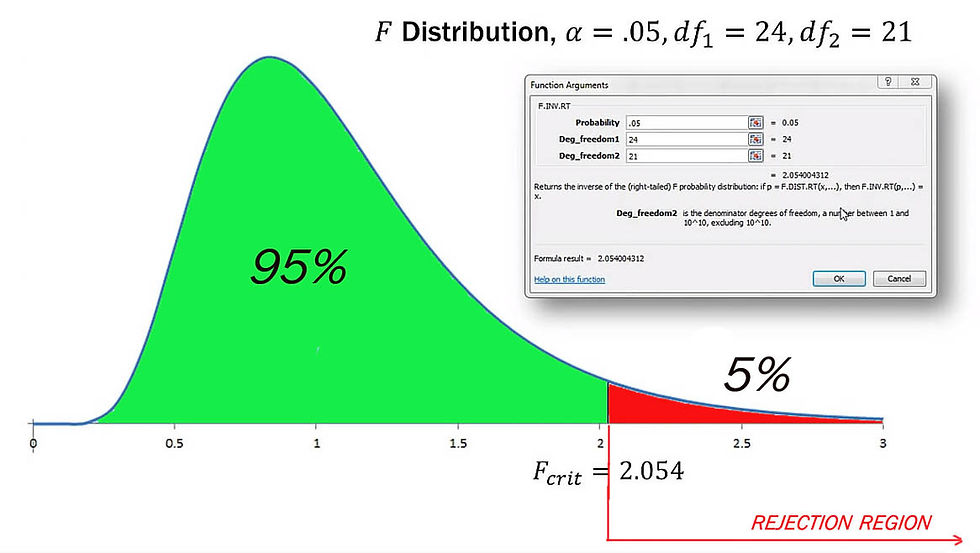top of page
Search
• Marek Vavrovic

# Hypothesis testing, F-Ratio, F-Distribution

When to use f-distribution?

In investing, variance is used to compare the relative performance of each asset in a portfolio. Because the results can be difficult to analyse, standard deviation is often used instead of variance. In either case, the case for the investor is to improve asset allocation. In investing, the variance of the returns among assets in a portfolio is analyzed as a means of achieving the best asset allocation. The variance equation, in financial terms, is a formula for comparing the performance of the elements of a portfolio against each other and against the mean.

You can use this test to find out if two machines are operating on the same level of variance. Which one is more predictable. If you compare two sport teams, you can find out which of them is more consistent in the scoring.

The F-ratio is a test statistic for multiple independent variables. It is used in ANOVA calculations and calculated as:

F-ratio = MSn / MSd

... where MS = SS / df = variance SS = Sum of the Squares df = degrees of freedom

MSn = Variance of sample 1.

MSd = Variance of sample 2.

MSn > MSd

## Using The F Statistic.

You can use the F statistic when deciding to support or reject the null hypothesis. In your F test results, you’ll have both an F value and an F critical value.

In general, if your calculated F value in a test is larger than your F statistic, you can reject the null hypothesis. However, the statistic is only one measure of significance in an F Test. You should also consider the p value. The p value is determined by the F statistic and is the probability your results could have happened by chance.

When Do I Reject the Null Hypothesis?

Reject the null when your p value is smaller than your alpha level. You should not reject the null if your critical f value is smaller than your F Value, unless you also have a small p-value.

EXAMPLE

The Happy Salmon company uses 2 machines to fill each 150g can of salmon. The Quality Manger wants to compare the variability, the variance, of the two canning machines.

? Standard deviation of machine 1 = standard deviation of machine 2 ?

To do this, a sample of cans is selected from each machine for testing. The results are as follow:

Sample size: n1=25 n2=22

Filling Weight: mean1 = 150.0592g mean2=149.9808g

Variance: Var(x1) = 0.1130 Var(x2)=0.0137

Standard deviation: s1=0.03361 g s2=0.1171 g

We are comparing the variances. If you look at these, the variance from machine 1 is slightly higher. These are samples. We do not know if this difference is statistically significant or it is related to sampling error. If you have significant result, it means that your results likely did not happen by chance.

The F-Ratio for population variances

We are not comparing the sample variance to a hypothesize variance. We are comparing 2 sample variances with each other. The easiest way to compare the relative size of two measurements is by using a ratio.The F-Distribution

When independent random samples n1 and n2, are taken from two normal populations with equal variances, the sampling distribution of the ration of those sample variances follows the F-distribution. The F-distribution is a distribution of ratios. To calculate the f-distribution we need to find degrees of freedom based on sample size:

df1 = 25 – 1 = 24

df2 = 22 – 1 = 21

To find the critical value for F-Distribution I use excel function:

F.INV.RT(Probability, Deg_freedom1, Deg_freedom2)

F.INV.RT(0.05, 24,21) = 2.054Set the test typeYou might think that based on the equal and not equal signs this should be a two-tailed distribution. However, remember that we always place the large sample variance in the numerator. Therefore, the F-ratio in this type of problem is always an upper-tailed test or distribution.

Calculating test statistic.The test statistic is the ratio of sample variances. If the test statistic is larger than the critical F-value, reject the null hypothesis.

Reject Ho: Based on samples, these variances are not equal.

F Test in Excel

A;P(F<=f) one-tail: This is p value. Reject the null when your p value is smaller than your alpha level.

B;